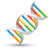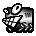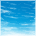# KEA128 BIT access

cancel
Showing results for
Did you mean:
SOLVED

## KEA128 BIT access

797 ViewsSenior Contributor I

Hi All,

I am using TRK- KEA128 Board and having following question regarding how to access individual bit of PORT.

Actually i am trying to read PORTA0 and PORTB3 here :in the following code  if Statement  work as expected (for checking logic High bit at PTA0 & PTB3) but as soon as i check the same code for logic low state (this is what i am doing in if statement  :   if  ((GPIOA_PDIR & GPIO_PDIR_PDI(0x0000)) >> 0 && (GPIOA_PDIR & GPIO_PDIR_PDI(0x0000)) >> 11) ) and the code always going in else statement , it doesn't matter PTA0 and PTB3 high or low.

for(;;) {

if  ((GPIOA_PDIR & GPIO_PDIR_PDI(0x0001)) >> 0 && (GPIOA_PDIR & GPIO_PDIR_PDI(0x0800)) >> 11)

{

GPIOA_PDOR =  0x30000;                                                                     // Initialise the PORTA, PORTB  LED PTC0 & PTC1

delay(65535);

}

else

{

GPIOA_PDOR =  0xC0000;                                                                    // LED PTC3 & PTC4

delay(65535);

}

}

return 0;

}

Thanks and Kind Regards,

Robin

1 Solution
351 ViewsSpecialist V

Hi

Yes, the naming convention used by the KE's ports is rather confusing. Essentially it is the same as all other Kinetis parts, being 32 bits in size, but the fact that it is referenced in documentation as 4 byte groups requires quite a large SW adaptation to use the same mnomenclature (used presumably to not scare off 8 bit users moving to the parts).

The code and the problem are however a bit confusing. Which code is working and which not? If I have interpreted correctly

if  ((GPIOA_PDIR & GPIO_PDIR_PDI(0x0001)) >> 0 && (GPIOA_PDIR & GPIO_PDIR_PDI(0x0800)) >> 11)

is a rather complicated way of writing

if (((GPIOA_PDIR & 0x0001) >> 0) && ((GPIOA_PDIR & 0x0800) >> 11))

which in in turn an unnecessarily shifted way to test

if (((GPIOA_PDIR & 0x0001) != 0) && ((GPIOA_PDIR & 0x0800) != 0))

which is probably simpler to write as

if ((GPIOA_PDIR & 0x0801) == 0x0801)

which I understand is however working correctly.

Is if  ((GPIOA_PDIR & GPIO_PDIR_PDI(0x0000)) >> 0 && (GPIOA_PDIR & GPIO_PDIR_PDI(0x0800)) >> 11)

then not working as expected?

If this is indeed the case then if ((GPIOA_PDIR & 0x0000) == 0x0801) can never be true, explaining the else case each time

and if ((GPIOA_PDIR & 0x0801) == 0x0000)

would be the simplest solution.

Regards

Mark

10 Replies
351 ViewsSenior Contributor III

Sorry, showing my assumptions again -- KEA departs from ALL other Kinetis in 'packing' 8-bit-like I/O ports into 32-bit registers...clearly just a confusing documentation-change to make it 'friendlier' to 8-bit users  -- all registers and controls are still 32-bit, as a block-of-four-ports, such that GPIOA_xx works on Ports DCBA, GPIOB_xx Ports HGFE...  So, just like you have it, function PortB3 is accessed as bit (1<<(8+3)), the '8' to shift into PortB.

May I suggest you check PORT control and confirm I/O pin mapping?.  And the state of GPIOA_PIDR, which defaults all I/O to 'read zero'.

You might also want to add another depth of parens -- I would want to insure the precedence of >> and &&.

352 ViewsSpecialist V

Hi

Yes, the naming convention used by the KE's ports is rather confusing. Essentially it is the same as all other Kinetis parts, being 32 bits in size, but the fact that it is referenced in documentation as 4 byte groups requires quite a large SW adaptation to use the same mnomenclature (used presumably to not scare off 8 bit users moving to the parts).

The code and the problem are however a bit confusing. Which code is working and which not? If I have interpreted correctly

if  ((GPIOA_PDIR & GPIO_PDIR_PDI(0x0001)) >> 0 && (GPIOA_PDIR & GPIO_PDIR_PDI(0x0800)) >> 11)

is a rather complicated way of writing

if (((GPIOA_PDIR & 0x0001) >> 0) && ((GPIOA_PDIR & 0x0800) >> 11))

which in in turn an unnecessarily shifted way to test

if (((GPIOA_PDIR & 0x0001) != 0) && ((GPIOA_PDIR & 0x0800) != 0))

which is probably simpler to write as

if ((GPIOA_PDIR & 0x0801) == 0x0801)

which I understand is however working correctly.

Is if  ((GPIOA_PDIR & GPIO_PDIR_PDI(0x0000)) >> 0 && (GPIOA_PDIR & GPIO_PDIR_PDI(0x0800)) >> 11)

then not working as expected?

If this is indeed the case then if ((GPIOA_PDIR & 0x0000) == 0x0801) can never be true, explaining the else case each time

and if ((GPIOA_PDIR & 0x0801) == 0x0000)

would be the simplest solution.

Regards

Mark

351 ViewsSenior Contributor I

Actually the code is not working for the following If Stament :

Not working:

if ((GPIOA_PDIR & GPIO_PDIR_PDI(0x0000)) >> 0 && (GPIOA_PDIR & GPIO_PDIR_PDI(0x0000)) >> 11) ).

working :

if ((GPIOA_PDIR & GPIO_PDIR_PDI(0x0001)) >> 0 && (GPIOA_PDIR & GPIO_PDIR_PDI(0x0800)) >> 11) ).

Thanks once again  for your suggestions

let me implement and check the changes you mentioned , will let you know the result guys.

Thanks and Regards,

Robin

351 ViewsSenior Contributor I

Thanks Mark,

The code is working fine with the following if Statement :

if (((GPIOA_PDIR & 0x0001) != 0) && ((GPIOA_PDIR & 0x0800) != 0))

One thing i would like to ask you, in above if Statement  i am doing the following changes

if (((GPIOA_PDIR & 0x0001) == 1) && ((GPIOA_PDIR & 0x0800) ==1))

which is same statement as above but don't know why the code is always going to else Statement ,whether it's PTA0 and PTB3 are low or high  . am i doing some mistake  here?

Sorry to ask you  such stupid question but m new to Cortex .

Thanks once again

MFG

Robin

351 ViewsSenior Contributor III

I would assume your reworked 'if' would need to be:

if (((GPIOA_PDIR & 0x0001) == 1) && ((GPIOA_PDIR & 0x0800) ==0x0800))

although, to make the intent 'more obvious':

if (((GPIOA_PDIR & (1<<0)) == (1<<0)) && ((GPIOA_PDIR & (1<<(8+3))) == (1<<(8+3))))

this way, you emphasize the 'bit position' of the port(s), the first being A0, and the second being 'B' (the 8 part of the shift count) plus the '3' of B3.

351 ViewsSpecialist V

Hi

I think that the main issues are logical coding mistakes (nothing KE or Cortex specific):

((GPIOA_PDIR & 0x0800) ==1)

will alwas be false.

It will be true if the bit corresponding to 0x0800 is '1' and when written as

((GPIOA_PDIR & 0x0800) ==0x0800)

or

((GPIOA_PDIR & 0x0800) !=0)

or

(GPIOA_PDIR & 0x0800)

Personally I avoid use of << (at least at the application level - maybe in some macros if they make sense) since it is quite easy to make mistakes with it (at least of you are as stupid as me) and I don't find that it makes code any more readable (but instead more cryptic).

For reading ports I use a macro which adapts itself to the processor type in question and so at the application level I would prefer something like

#define TEST_INPUT   KE_PORTB_BIT3 // give the input a meaningful name

if (_READ_PORT_MASK(A, TEST_INPUT) != 0) { // check whether the port input is '1'

which I think makes the itentions clear, hides any cryptic details and also is portable between processors.

In the case of all Kinetis processors the macro is simply:

Regards

Mark

For the complete "out-of-the-box" Kinetis experience and faster time to market

351 ViewsSenior Contributor III

Hi Mark!

I think we can agree to disagree on constant forms!

After 40 years of embedded programming:

0x0800

0b0000100000000000

1<<11

are all OBVIOUSLY the same number, and I can freely see the correspondence.

That being said, I believe the 1<<11 form 'most obviously states' the bit-offset nature of the mask.  Now in this particular case, due to 'some weirdo' at Freescale who decided to make this whole '8 bit subport stuff' (and still use GPIOB, which now no LONGER relates to Port 'B"!) it makes sense to make it easier to emphasize the 'sub port' indexing.  I would certainly vote for a "#define PORTB 8" , and then make this constant 1<<(PORTB + 3) to clearly indicate bit PortB3.  I understand your particular circumstances, most especially the need to support EVERY Kinetis with a 'minimum' of overhead, but I would find remembering the macro name (and contents) more difficult.

Of course the IAR compiler won't use these 'constants' at ALL to test bits -- what it will actually do is shift the bit in question to the sign-bit (so left 20 in this case!), and test THAT (BPL/BMI) for the zero/non-zero implication of the boolean statement.

 if (((GPIOA_PDIR & (1<<0)) == (1<<0)) && ((GPIOA_PDIR & (1<<(8+3))) == (1<<(8+3))))

??main_0:

 0x416c: 0x48c9 LDR.N R0, ??DataTable0_4 ; GPIOA_PDIR 0x416e: 0x6800 LDR R0, [R0] 0x4170: 0x07c0 LSLS R0, R0, #31 0x4172: 0xd507 BPL.N ??main_1 ; 0x4184 0x4174: 0x48c7 LDR.N R0, ??DataTable0_4 ; GPIOA_PDIR 0x4176: 0x6800 LDR R0, [R0] 0x4178: 0x0500 LSLS R0, R0, #20 0x417a: 0xd503 BPL.N ??main_1 ; 0x4184

Loads GPIOA_PDIR twice in this case because it is defined 'volatile'.

351 ViewsSpecialist V

Hi Earl

I prefer to not get involved too much in issues of coding "styles" rather than coding "correctness" since it is a bit like discussion religion (but hopefully not leading to any deaths and war).

#define KE_PORTB_BIT3 0x00000800

#define KE_PORTB_BIT3 0b0000100000000000

#define KE_PORTB_BIT3 (1 << 11)

are of course the same and a coder's preference.

I like to simply use the KE_PORTB_BIT3 at the application level as a mask to keep consistent and in fact don't really think of it as a bit or mask when using it but rather simply as a name that one can assume is a correct reference.

The final assembler code is identical in each case, however written.

Where I can certainly agree/confirm is that it would have been simpler from a programmer's point of view if the ports on the KE were not used as pseudo 8 bit ones in the documentation since the nasty bit in the macro above is that one needs to use

when the TEST_INPUT is on KE ports A, B, C or D

and then

if it is on KE port E, F, G or H

This needs a little attention since the underlying macro can't accept an intermediate define (such as allowing _READ_PORT_MASK(KE_PORT_F, TEST_INPUT)

But since it is limited to this it is not the end of the world. The actual KE operation with port MUX control based on peripheral functions and their priorities, rather than a PORTx_PCRy, causes more headaches, especially when simulating the behaviour!).

Regards

Mark

For the complete "out-of-the-box" Kinetis experience and faster time to market

351 ViewsSenior Contributor III

We Are Right!:smileyshocked:

351 ViewsSenior Contributor I

Hello All,

Thanks alot , now i got it.

Thanks n Kind Regards,

Robin